# Advanced information about circles

##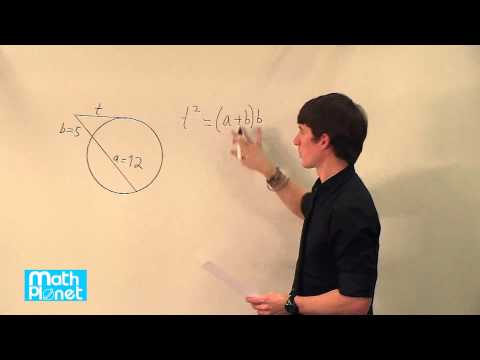By MathPlanetVideos

Find the value of t in the figure# Basic information about circles

##By MathPlanetVideos

What's the angle of the circle arc if we divide a cicle in 12 equally sized pieces# 11.6 Areas of Circles, Sectors & Segments (Lesson)

##By AutenMath

A lesson on finding the area of circles, sectors and segments# Partitioning rectangles, squares, and circles into halves and fourths-2nd grade

##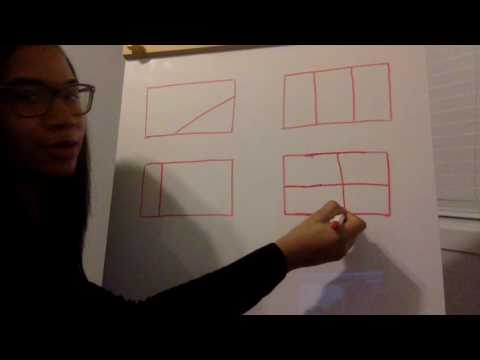By Tyris Hall

Partitioning rectangles into halves# Partitioning Circles and Rectangles: 2.G.3

##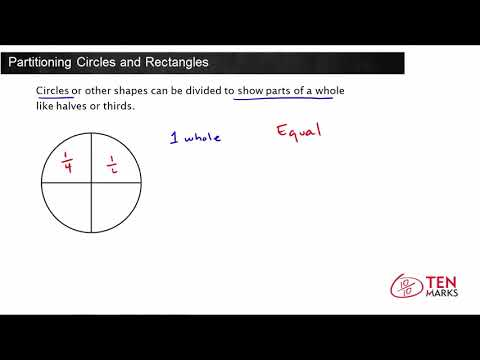By Tenmarks Amazon

Students learn to create and discuss halves, thirds, and fourths of circles and rectangles.# Partitioning Circles and Rectangles: 2.G.3

##By Tenmarks Amazon

Students learn to create and discuss halves, thirds, and fourths of circles and rectangles.# Circles

##By Udacity

YouTube presents Circles, an educational video resource on math.# Math-U-See Geometry - Homeschooling Help Circles Inscribed Angles - TabletClass.com

##By TabletClass Math

Youtube Presents Math-U-See Geometry - Homeschooling Help Circles Inscribed Angles - TabletClass.com an educational video resources on math# Equation for a circle using the Pythagorean Theorem | Circles | Geometry | Khan Academy

##By Khan Academy

Equation for a circle using the Pythagorean Theorem | Circles | Geometry | Khan Academy# Pythagorean theorem and the equation of a circle

##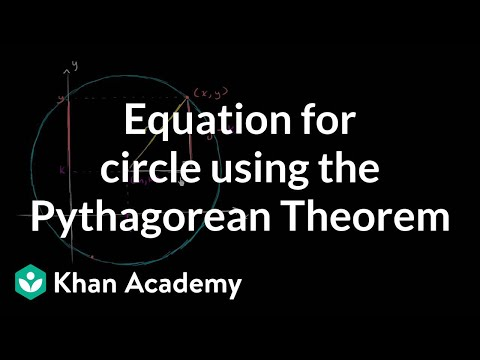By Khan Academy

Pythagorean theorem and the equation of a circle# Pythagorean theorem and the equation of a circle

##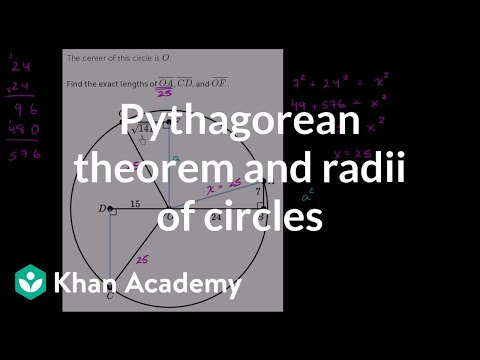By Khan Academy

Pythagorean theorem and the equation of a circle# Tangents and Circles - YourTeacher.com - Geometry Help

##By yourteachermathhelp

Students learn the following theorems related to tangents. If a line is tangent to a circle, then the line is perpendicular to the radius at the point of tangency. If a line is perpendicular to a radius at its outer endpoint, then the line is tangent to the circle. If two tangent segments are drawn to a circle from an external point, then the tangent segments are congruent. Students are then asked to use these theorems to find missing segment lengths and missing angle measures in given figures. Students also learn the definitions of common internal tangents and common external tangents.# Proof: Right triangles inscribed in circles | High School Math | Khan Academy

##By Khan Academy

Proof showing that a triangle inscribed in a circle having a diameter as one side is a right triangle. All Khan Academy content is available for free at www.khanacademy.org# Area of inscribed equilateral triangle (some basic trig used) | Circles | Geometry | Khan Academy

##By Khan Academy

This video will give you a good feel for what inscribed figures are like. It uses some trigonometry to figure out what the area of an equilateral triangle inscribed in a circle is, given the circle's radius. NOTE: We don't actually need trigonometry to solve this problem. Notice that we can view the equilateral triangle as six identical 30-60-90 triangles, the hypotenuses of which are radii of the circle, then use the 30-60-90 triangle side length ratios to figure out the rest of what we need to know to solve the problem.# Area of inscribed equilateral triangle (some basic trig used) | Circles | Geometry | Khan Academy

##By Khan Academy

This video will give you a good feel for what inscribed figures are like. It uses some trigonometry to figure out what the area of an equilateral triangle inscribed in a circle is, given the circle's radius. NOTE: We don't actually need trigonometry to solve this problem. Notice that we can view the equilateral triangle as six identical 30-60-90 triangles, the hypotenuses of which are radii of the circle, then use the 30-60-90 triangle side length ratios to figure out the rest of what we need to know to solve the problem.# Inscribing and circumscribing circles on a triangle

##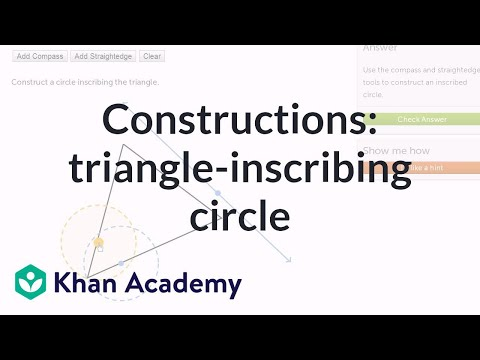By Khan Academy

Inscribing and circumscribing circles on a triangle# Circles: radius, diameter, circumference and Pi | Geometry | Khan Academy

##By Khan Academy

The radius is the distance between the center and any point on the circumference of a circle. The diameter is always twice the radius. The circumference is the perimeter of the circle.# Circle: Application: Finding Area and Length of a Race Track

##By easymathk12

An oval track is made by erecting semicircles on each end of a 60m by 120m rectangle. Find the length of the track and the area enclosed by the track.